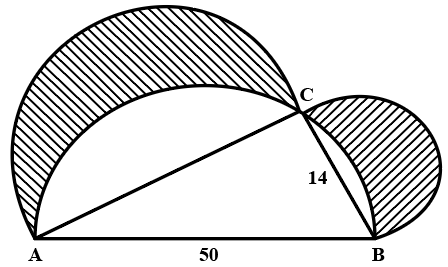Question 11

# In a figure, $$\triangle ABC$$ is a right angled triangle at $$C$$, semicircles are drawn on $$AC$$, $$BC$$ and $$AB$$'s diameter. Find the area of the shaded region.Note: Figure not as per scale

Solution

Area of shaded region = area of semicircle on BC + area of semicircle of AC - area of unshaded semicircle regions
Area of unshaded semicircle regions = area of semicircle on AB - area of triangle ABC
Given,
AB = 50, BC = 14
In triangle ABC, $$AC^2+BC^2 = AB^2$$
Solving we get,
AC = 48
Area of unshaded semicircle regions = $$\ \frac{\pi\ \left(25\right)^2}{2}\ -\frac{1}{2}\times\ 48\times\ 14=\frac{625\pi\ }{2}-336$$
Area of shaded region = $$\frac{576\pi\ }{2}\ +\frac{49\pi}{2}\ -\frac{625\pi}{2}\ +336$$ = 336